# How to add and subtract dates, days, weeks, months and years in Excel

In this tutorial, you will find a variety of useful formulas to add and subtract dates in Excel, such as subtracting two dates, adding days, weeks, months and years to a date, and more.

If you have been following our tutorials to working with dates in Excel, you already know an array of formulas to calculate different time units such as days, weeks, months and years.

When analyzing the date information in your worksheets, you are likely to perform some arithmetic operations with those dates too. This tutorial explains a few formulas for adding and subtracting dates in Excel that you may find useful.

## How to subtract dates in Excel

Supposing you have two dates in cells A2 and B2, and now you want to subtract one date from the other to know how many days are between these dates. As is often the case in Excel, the same result can be achieved in several ways.

#### Example 1. Subtract one date from the other directly

As you probably know, Microsoft Excel stores each date as a unique serial numbers beginning with 1 that represents January 1, 1900. So, you are actually subtracting two numbers, and an ordinary arithmetic operation works without a hitch:

`=B2-A2`

#### Example 2. Subtract dates using Excel DATEDIF function

If the above formula looks too plain, you can achieve the same result in a guru-like way by using Excel's DATEDIF function:

`=DATEDIF(A2, B2, "d")`

The following screenshot demonstrates that both calculations return identical results, except for row 4 where the DATEDIF function returns the #NUM error. Let's figure out why that happens.

When you subtract a more recent date (6-May-2015) from an earlier date (1-May-2015), the subtraction operation returns a negative number (-5) exactly as it should. The syntax of the Excel DATEDIF function, however, does not allow the start date to be greater than the end date and therefore it returns an error.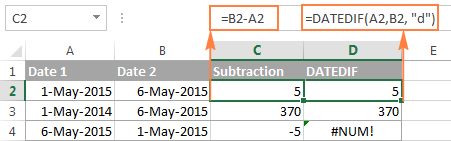#### Example 3. Subtract a date from the current date

To subtract a date from today's date, you can employ either of the above formulas. Just use the TODAY() function instead of date 1:

`=TODAY()-A2`

or

`=DATEDIF(A2,TODAY(), "d")`

Like in the previous example, both formulas work fine when today's date is greater than the date you are subtracting from it, otherwise DATEDIF fails: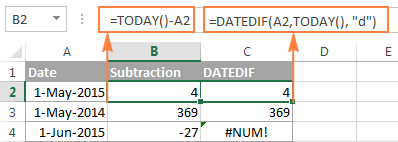#### Example 4. Subtracting dates with Excel DATE function

If you prefer to supply the dates directly in the formula, then enter each date using the DATE(year, month, day) function and then subtract one date from the other.

For instance, the following formula subtracts 15-May-2015 from 20-May-2015 and returns the difference of 5 days:

`=DATE(2015, 5, 20) - DATE(2015, 5, 15)`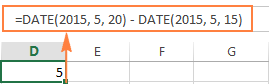Wrapping up, when it comes to subtracting dates in Excel and you want to find out how many days are between two dates, it makes sense to go with the easiest and most obvious option - simply subtract one date directly from another.

If you are looking to count the number of months or years between two dates, then the DATEDIF function is the only possible solution and you will find a few formula examples in the next article that will cover this function in full details.

Now that you know how to subtract two dates, let's see how you can add or subtract days, months, or years to a given date. There are a number of Excel functions suitable for this purpose, and which one you use depends on which unit you want to add or subtract.

## How to subtract or add days to date in Excel

If you have a date in some cell or a list of dates in a column, you can add or subtract a certain number of days to those dates using a corresponding arithmetic operation.

#### Example 1. Adding days to a date in Excel

The general formula to add a specified number of days to a date in as follows:

Date + N days

The date can be entered in several ways:

• As a cell reference, e.g. `=A2 + 10`
• Using the DATE(year, month, day) function, e.g. `=DATE(2015, 5, 6) + 10`
• As a result of another function. For example, to add a given number of days to the current date, use the TODAY() function: `=TODAY()+10`

The following screenshot demonstrates the above formulas in action. The current date at the moment of writing was 6 May, 2015: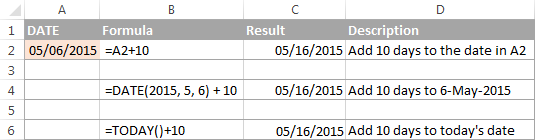Note. The result of the above formulas is a serial number representing the date. To get it displayed as a date, select the cell(s) and press Ctrl+1 to open the Format Cells dialog. On the Number tab, select Date in the Category list, and then choose the date format you want. For the detailed steps, please see How to change date format in Excel.

#### Example 2. Subtracting days from a date in Excel

To subtract a given number of days from a certain date, you perform a usual arithmetic operation again. The only difference from the previous example is that you type the minus sign instead of plus :)

Date - N days

Here are a few formula examples:

• `=A2-10`
• `=DATE(2015, 5, 6)-10`
• `=TODAY()-10`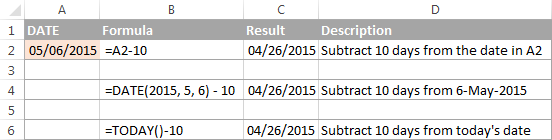## How to add or subtract weeks to date

In case you want to add or subtract whole weeks to a certain date, you can use the same formulas as for adding / subtracting days, and simply multiply the number of weeks by 7:

Adding weeks to a date in Excel:

cell+ N weeks * 7

For example, you add 3 weeks to the date in A2, use the following formula: `=A2+3*7`.

Subtracting weeks from date in Excel:

cell - N weeks * 7

To subtract 2 weeks from today's date, you write `=TODAY()-2*7`.

## How to add / subtract months to date in Excel

If you want to add or subtract a certain number of whole months to a date, you can employ either the DATE or EDATE function, as demonstrated below.

#### Example 1. Add months to a date with Excel DATE function

Taking a list of dates in column A for example, type the number of dates you want to add (positive number) or subtract (negative number) in some cell, say C2.

Enter the following formula in cell B2 and then drag the corner of the cell all the way down to copy the formula to other cells:

`=DATE(YEAR(A2), MONTH(A2) + \$C\$2, DAY(A2))`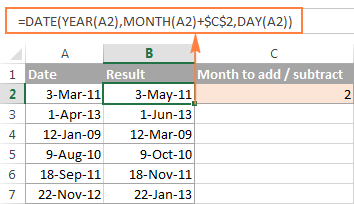Now, let's see what the function is actually doing. The logic behind the formula is obvious and straightforward. The DATE(year, month, day) function takes the following arguments:

• the year of the date in cell A2;
• the month of the date in A2 + the number of months you specified in cell C2, and
• the day of the date in A2.

Yep, it's that simple :) If you type a negative number in C2, the formula will subtract months instead of adding them: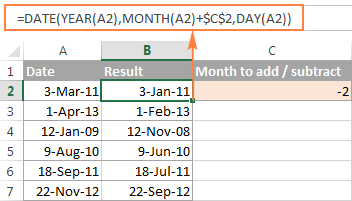Naturally, nothing prevents you from typing the minus sign directly in the formula to subtract months from a date:

`=DATE(YEAR(A2), MONTH(A2) - \$C\$2, DAY(A2))`

And of course, you can type the number of month to add or subtract in the formula instead of referring to a cell:

`=DATE(YEAR(date), MONTH(date) + N months, DAY(date))`

The real formulas could look similar to these:

• Add months to date: `=DATE(YEAR(A2), MONTH(A2) + 2, DAY(A2))`
• Subtract months from date: `=DATE(YEAR(A2), MONTH(A2) - 2, DAY(A2))`

#### Example 2. Add or subtract months to a date with Excel EDATE

Microsoft Excel provides a special function that returns a date that is a specified number of months before or after the start date - the EDATE function. It is available in modern versions of Excel 2007, 2010, 2013 and upcoming Excel 2016.

In your `EDATE(start_date, months)` formulas, you supply the following 2 arguments:

• Start_date - the start date from which to count the number of months.
• Months - the number of months to add (a positive value) or subtract (a negative value).

The following formula used on our column of dates yields exactly the same results as the DATE function in the previous example: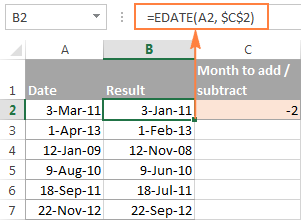When using the EDATE function, you can also specify the start date and the number of month to add / subtract directly in the formula. Dates should be entered by using the DATE function or as results of other formulas. For example:

• To add months in Excel:

`=EDATE(DATE(2015,5,7), 10)`

The formula adds 10 months to 7-May-2015.

• To subtract months in Excel:

`=EDATE(TODAY(), -10)`

The formula subtracts 10 months from today's date.

Note. The Excel EDATE function returns a serial number representing the date. To force Excel to display it as a date, you should apply the Date format to the cells with your EDATE formulas. Please see Changing the date format in Excel for the detailed steps.

## How to subtract or add years to date in Excel

Adding years to a date in Excel is done similarly to adding months. You use the DATE(year, month, day) function again, but this time you specify how many years you want to add:

DATE(YEAR(date) + N years, MONTH(date), DAY(date))

In your Excel worksheet, the formulas may look as follows:

• To add years to a date in Excel:

`=DATE(YEAR(A2) + 5, MONTH(A2), DAY(A2))`

The formula adds 5 years to the date in cell A2.

• To subtract years from a date in Excel:

`=DATE(YEAR(A2) - 5, MONTH(A2), DAY(A2))`

The formula subtracts 5 years from the date in cell A2.

If you type the number of year to add (positive number) or subtract (negative number) in some cell and then refer to that cell in the DATE function, you will get a universal formula: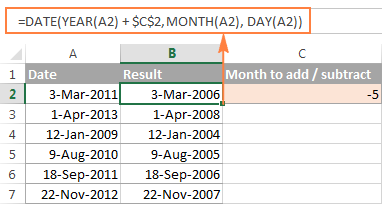## Add / subtract days, months and years to date

If you carefully observed the two previous examples, I think you have already guessed how to add or subtract a combination of years, months and days to a date in a single formula. Yep, using the good old DATE function :)

DATE(YEAR(date) + X years, MONTH(date) + Y months, DAY(date) + Z days)

To subtract years, months, days:

DATE(YEAR(date) - X years, MONTH(date) - Y months, DAY(date) - Z days)

For example, the following formula adds 2 years, 3 months and subtracts 15 days from a date in cell A2:

`=DATE(YEAR(A2) + 2, MONTH(A2) + 3, DAY(A2) - 15)`

Applied to our column of dates, the formula takes the following shape:

`=DATE(YEAR(A2) + \$C\$2, MONTH(A2) + \$D\$2, DAY(A2) + \$E\$2)`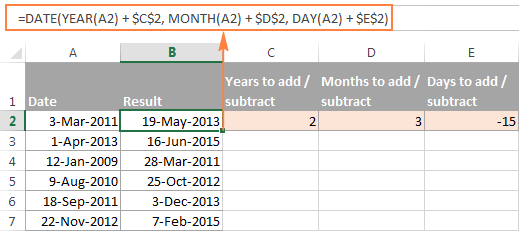## How to add and subtract times in Excel

In Microsoft Excel, you can add or subtract times using the TIME function. It lets you operate on time unites (hours, minutes and seconds) exactly in the same way as you handle years, months and days with the DATE function.

cell + TIME(hours, minutes, seconds)

To subtract time in Excel:

cell - TIME(hours, minutes, seconds)

Where A2 contains the time value you want to change.

For example, to add 2 hours, 30 minutes and 15 seconds to the time in cell A2, you can use the following formula:

`=A2 + TIME(2, 30, 15)`

If you want to add and subtract time unites within one formula, just add the minus sign to the corresponding values:

`=A2 + TIME(2, 30, -15)`

The above formula adds 2 hours and 30 minutes to the time in cell A2 and subtracts 15 seconds.

Alternatively, you can enter the time unites you want to changes in some cells, and refer to those cells in your formula:

`=A2 + TIME(\$C\$2, \$D\$2, \$E\$2)`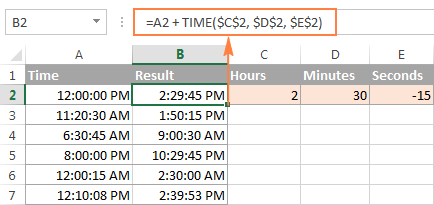If the original cells contain both date and time, the above formula works perfectly too: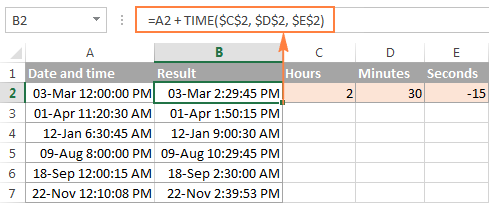## Date & Time Formula Wizard - quick way to add and subtract dates in Excel

Now that you know a bunch of different formulas to calculate dates in Excel, wouldn't you want to have just one that can do all this? Of course, such a formula can never exist. However, there exists the Date & Time Wizard that can build any formula for you on the fly, provided that you have our Ultimate Suite installed in your Excel. Here's how:

1. Select the cell in which you want to insert the formula.
2. Head to the Ablebits Tools tab, and click the Date & Time Wizard button:3. The Date & Time Wizard dialog window shows up. Depending on whether you want to add or subtract dates, switch to the corresponding tab, supply data for the formula arguments, and click the Insert Formula button.

As an example, let's add a few months to the date in cell A2. For this, you go to the Add tab, type A2 in the Enter a date box (or click in the box and select the cell on the sheet), and type the number of months to add in the Month box.

The wizard makes a formula and shows its preview in the cell. It also shows the calculated date under Formula result: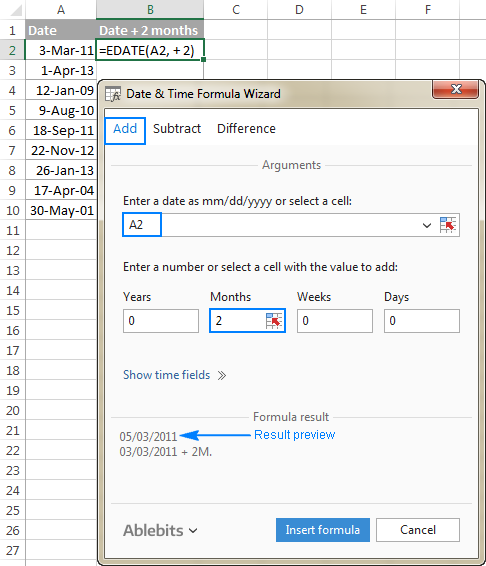If you are satisfied with the result, click the Insert formula button. Once the formula is added, you can copy it to as many cells as necessary: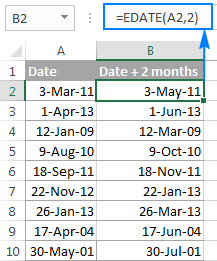That was quite a simple formula, wasn't it? Let's give the wizard something more challenging to work on. For example, let us subtract some years, months, weeks and days from the date in A2. To have it done, switch to the Subtract tab and type the numbers in the corresponding boxes. Or you can enter the units in separate cells and supply references to those cells, as shown in the screenshot below: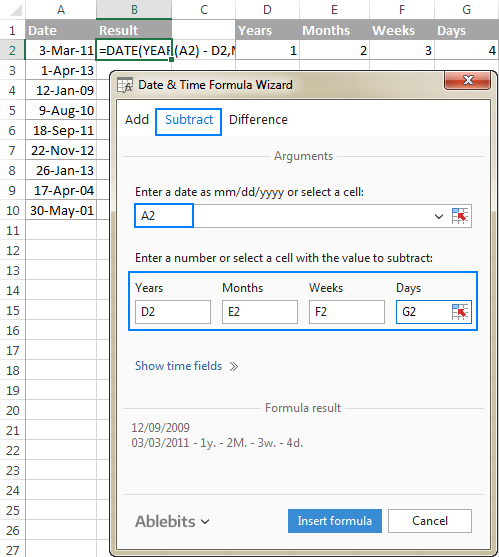Clicking the Insert formula button inputs the following formula in A2:

`=DATE(YEAR(A2)-D2,MONTH(A2)-E2,DAY(A2)-G2-F2*7)`

If you plan to copy the formula to other cells, you have to change all cell references except A2 to absolute references so that the formula copies correctly (by default, the wizard always uses relative references). To fix the reference, you simply type the \$ sign before the row and column coordinates, like this:

`=DATE(YEAR(A2)-\$D\$2,MONTH(A2)-\$E\$2,DAY(A2)-\$G\$2-\$F\$2*7)`

And get the following results: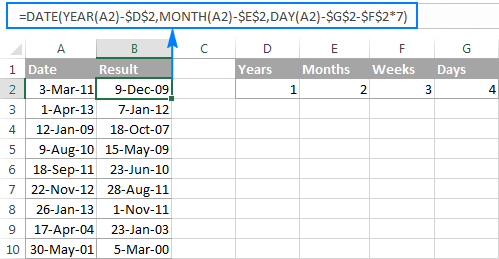Additionally, you can click the Show time fields link and add or subtract date and time units with one formula.

If you wish to play with the Date & Time Formula Wizard in your own worksheets, you are welcome to download the 14-day trial version of the Ultimate Suite.

This is how you add and subtract dates in Excel. I am hopeful you have learned a couple of useful functions today. I thank you for reading and hope to see you on our blog next week.